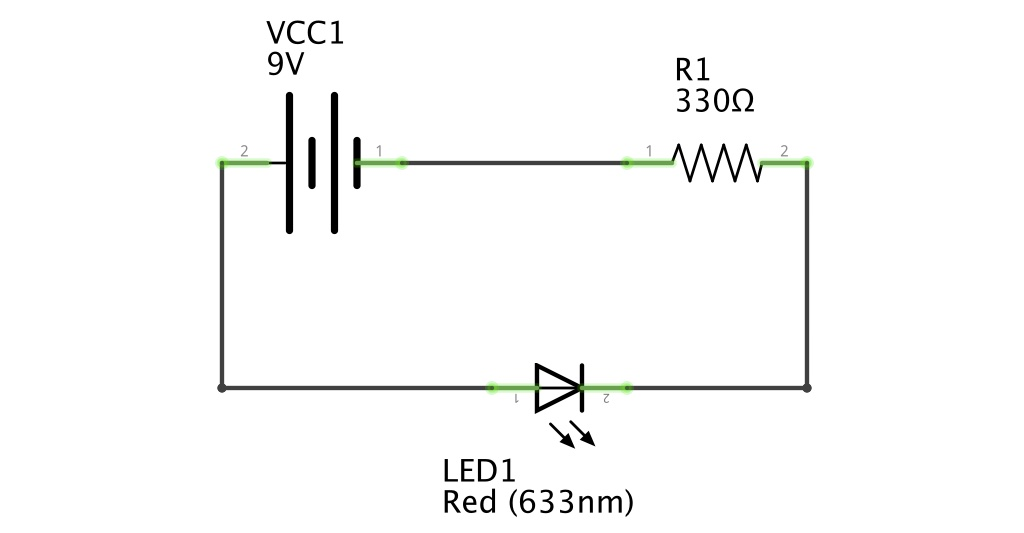## Kirchhoff's LawTo understand how resistors are used, we need to introduce you to an important concept, “Kirchhoff's Voltage Law":

V (Source) = V (Resistor) + V (LED)

This law basically states that the sum of all voltage across the circuit is equal to the source voltage.

But when we are talking about the voltage of a resistor or an LED, what exactly are we referring to? We are actually talking about the component's voltage potential.

What's important to understand in electronics (basic to advanced) is that every component on the circuit has a voltage potential. A voltage potential is the amount of energy used to push current through the component. Just imagine a number of soccer balls trying to squeeze through a small pipe. The amount of energy needed to push the soccer balls through the pipe is the pipe's "Voltage Potential".

But what's providing the energy/voltage? The battery of course! Kirchhoff's Law further states that the sum of the Voltage Potentials (or Volts) of the components cannot be more than the Voltage Source (9V Battery), otherwise there isn't enough energy to push current through the components.

But how do we know the voltage potential of a component? Many basic components, such as the resistor or the capacitor (we'll cover that one soon) simply follow Kirchhoff's Voltage Law. That is, if we have a battery and a resistor, the voltage potential of the resistor will be:

9V = V (Resistor), Resistor Voltage is 9V.

But what if we had two resistors? If the resistors are in series, we will need to add the resistors' value together and calculate the current first (we'll do that later on).

Looking at the schematic above, powered with a 9V battery source, our circuit now has two components, a resistor and an LED.

The LED has its own voltage potential and looking up at the "forward voltage" of the LED in its data sheet, we'll get a voltage of 2V.

So, using Kirckhoff's law, our equation goes:

9V (battery)= V (Resistor) + 2V (LED)

V (resistor) = 7